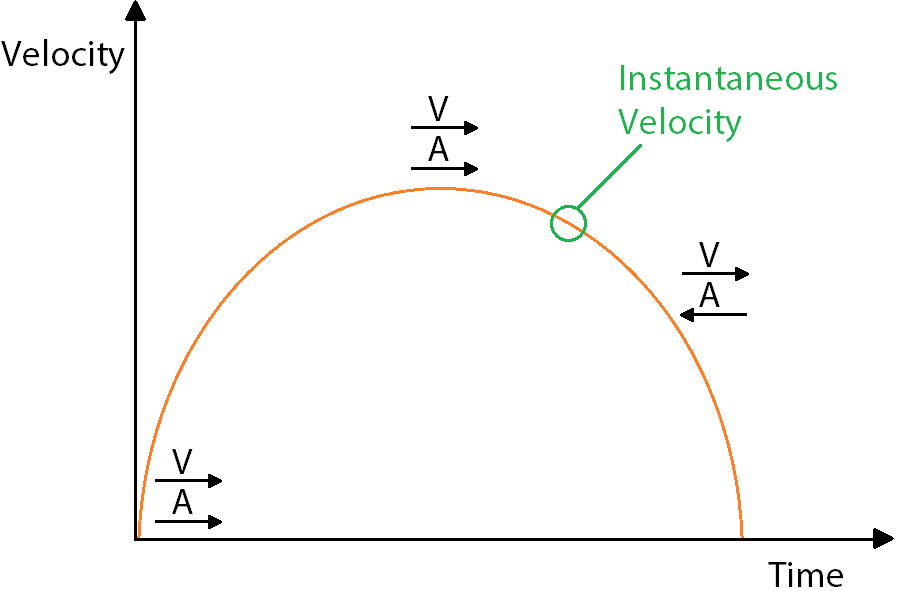# What is the Difference Between Velocity and Acceleration?

Velocity is the rate of motion, or speed, in a specific direction. This means that velocity is a vector. We need both its magnitude, or value, and its direction. If we only know the speed, that is not the velocity.

We have to also know the direction. If we just know we’re going 30 miles an hour, that is only our speed. If we know were going 30 miles an hour in a southwest direction, then our velocity is 30 miles an hour southwest. That is a vector.

## Average Velocity

Average velocity is one of the two velocities that you need to keep in mind. Average velocity is found by dividing the distance traveled by the time traveled. Your formula would be velocity equals distance over time. You may start slow, speed up, and then slow down as you come to your destination. From point A to point B how far you traveled is going to be your distance. Let’s say you traveled 120 miles and you were traveling southwest.

It took you 60 minutes to get there. You would divide that. 120 divided by 60 equals 2. You were going two miles per minute southwest. That would be your velocity. Two miles per minute southwest. Now, if we were trying to do that in hours, we could have just changed it to one hour. That would have been 120 miles per hour. That is really fast and not a likely velocity, but if something was going that fast, its velocity would be 120 miles per hour southwest or two miles per minute southwest.

It’s important to include the direction, otherwise you only have speed. The fact that you have direction is what makes it velocity. Your average velocity is how far you traveled divided by how long it took overall for the whole trip. Your velocity is constantly increasing and decreasing as you are traveling.

The other type of velocity to look for is instantaneous velocity.

## Instantaneous Velocity

This refers to an object’s velocity at an exact second in time and usually requires the use of calculus to be determined. If you hit a pothole, it would be good to know if you were going one mile an hour or 60 miles an hour. Knowing at that exact second time how fast you were going and what direction, that would be important. Sometimes you might have to find instantaneous velocity, but most commonly average velocity is what you hear about.

Now, acceleration is the change in the velocity of an object. Acceleration is also a vector and will have both magnitudes, a value, and direction. Remember, we talked about your velocity would be increasing and decreasing as you traveled. Well, acceleration is the change in that velocity. Acceleration points the same direction as velocity while you are maintaining or increasing speed. Velocity is going this direction.

Acceleration is going this direction while you are maintaining or increasing speed. Deceleration is another word for acceleration when you are losing the speed, when your velocity is decreasing. Deceleration is going to point in the opposite direction as velocity. If you’re going this direction, but you’re slowing down, then velocity still points this way. You’re still traveling this way, but your acceleration is decreasing. Your velocity is decreasing. When you’re decreasing speed, acceleration is going to go in the opposite direction of velocity. Your velocity is always going to stay the same way, because you’re going to be traveling forward in time.

Now, deceleration is still referred to as acceleration, because, remember, acceleration is a vector with magnitude and direction. It’s going to point backward, opposite a velocity, whenever you’re decreasing speed, or decelerating.

It’s going to point forward in the same direction as velocity whenever you’re maintaining or increasing speed. Sometimes the word deceleration is used. Sometimes it’s not. Sometimes it’s just acceleration both ways. It’s important to know that it can still be called acceleration, even whenever you’re decreasing speed. This is the way that it would look if it was represented with vectors. Now, let’s look at our example over here.You’ve got velocity over time here. This arc is your distance. You start out with a low velocity and build up. Then you slow down as you come to your destination at the end. You can see your velocity is still going in this direction, increasing, and your acceleration is increasing in this direction. Up at the top, velocity and acceleration are both pointing in the same direction.

Then, as you start to slow down, velocity is still going to go in the same direction, because you were moving forward in time. Even while you’re slowing down, velocity is going to point in the same direction moving forward. Acceleration points backward now, because you are slowing down. As your velocity decreases, acceleration is going to point opposite of the velocity.

This will be your distance covered (this whole line), and you would divide by this time here to find your average velocity. Instantaneous velocity would be if you said, “Right here at this point I want to know what my velocity was.” Velocity is your rate of speed in a specific direction. Your average velocity is found by dividing the distance you’ve covered over the time it took you to cover it.

Instantaneous velocity refers to an object’s velocity in an exact moment in time. Acceleration is the change in the velocity of an object, either as it increases or decreases. Acceleration is also a vector and will have both a value and a direction. It will face the same direction as your velocity if you are increasing speed, and it will face the opposite direction if you are decreasing speed.